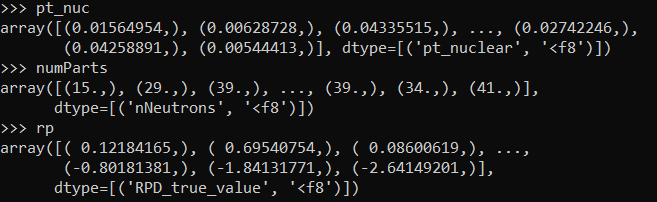# PyROOT giving wrong first value

Hello,

I’m currently working on putting together an array2tree method for my codebase to replace the now deprecated root_numpy method, and have come up with a brute-force method that mostly works:

``````def array2tree(arr, tree = None):
if tree == None:
tree = TTree("tree","tree")
b = tree.Branch(arr.dtype.names,arr, 'normal/D')
for i in range(len(arr)):
arr = arr[i]
tree.Fill()

return tree
``````

When I pass a Numpy array to this method, however, the first (and only the first) value of the array is affected. For reference, the sample dataset I’m testing on has the following values in the TTree generated:

While the associated Numpy arrays have values:I’m suspecting there’s some strange wrapping happening here, but I’m not sure what’s causing it.

_ROOT Version: 6.24/06
Platform: Linux
Compiler: Not Provided

I’m going to close this one myself, it was a Pandas → Numpy conversion problem and not pertinent to ROOT at all.

Dear @LittleRoot ,

Thanks for reaching out, I’m glad you could solve your initial issue so quickly!

Let me take this chance to tell you that through `RDataFrame`, you can convert numpy arrays to TTree very simply (example taken from the docs):

``````import ROOT
import numpy
# Read data from NumPy arrays
# The column names in the RDataFrame are taken from the dictionary keys
x, y = numpy.array([1, 2, 3]), numpy.array([4, 5, 6])
df = ROOT.RDF.FromNumpy({"x": x, "y": y})

# Use RDataFrame as usual, e.g. write out a ROOT file
df.Define("z", "x + y").Snapshot("tree", "file.root")
``````

The above snippet assumes latest ROOT version 6.28.

Hope this helps,
Vincenzo

This topic was automatically closed 14 days after the last reply. New replies are no longer allowed.